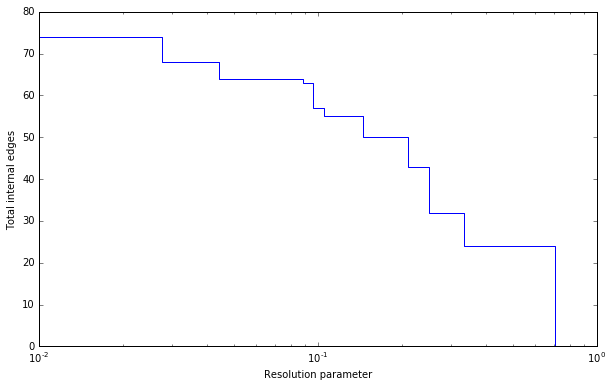The basic interface explained in the Introduction should provide you enough to start detecting communities. However, perhaps you want to improve the partitions further or want to do some more advanced analysis. In this section, we will explain this in more detail.

## Optimiser¶

Although the package provides simple access to the function find_partition(), there is actually an underlying Optimiser class that is doing the actual work. We can also explicitly construct an Optimiser object:

>>> optimiser = louvain.Optimiser()


The function find_partition() then does nothing else then calling optimise_partition() on the provided partition.

>>> diff = optimiser.optimise_partition(partition)


But optimise_partition() simply tries to improve any provided partition. We can thus try to repeatedly call optimise_partition() to keep on improving the current partition:

>>> G = ig.Graph.Erdos_Renyi(100, p=5./100)
>>> partition = louvain.ModularityVertexPartition(G)
>>> improv = 1
>>> while improv > 0:
...   improv = optimiser.optimise_partition(partition)


Even if a call to optimise_partition() did not improve the current partition, it is still possible that a next call will improve the partition. Of course, if the current partition is already optimal, this will never happen, but it is not possible to decide whether a partition is optimal.

The optimise_partition() itself is built on a basic algorithm: move_nodes(). You can also call this function yourself. For example:

>>> diff = optimiser.move_nodes(partition)


The usual strategy in the Louvain algorithm is then to aggregate the partition and repeat the move_nodes() on the aggregated partition. We can easily repeat that:

>>> partition = louvain.ModularityVertexPartition(G)
>>> while optimiser.move_nodes(partition) > 0:
...   partition = partition.aggregate_partition()


This summarises the whole Louvain algorithm in just three lines of code. Although this finds the final aggregate partition, this leaves it unclear the actual partition on the level of the individual nodes. In order to do that, we need to update the membership based on the aggregate partition, for which we use the function from_coarse_partition().

>>> partition = louvain.ModularityVertexPartition(G)
>>> partition_agg = partition.aggregate_partition()
>>> while optimiser.move_nodes(partition_agg):
...   partition.from_coarse_partition(partition_agg)
...   partition_agg = partition_agg.aggregate_partition()


Now partition_agg contains the aggregate partition and partition contains the actual partition of the original graph G. Of course, partition_agg.quality() == partition.quality() (save some rounding).

The function move_nodes() in turn relies on two key functions of the partition: diff_move() and move_node(). The first calculates the difference when moving a node, and the latter actually moves the node, and updates all necessary internal administration. The move_nodes() then does something as follows

>>> for v in G.vs:
...   best_comm = max(range(len(partition)),
...                   key=lambda c: partition.diff_move(v.index, c))
...   partition.move_node(v.index, best_comm)


The actual implementation is more complicated, but this gives the general idea.

## Resolution profile¶

Some methods accept so-called resolution parameters, such as CPMVertexPartition or RBConfigurationVertexPartition. Although some method may seem to have some ‘natural’ resolution, in reality this is often quite arbitrary. However, the methods implemented here (which depend in a linear way on resolution parameters) allow for an effective scanning of a full range for the resolution parameter. In particular, these methods somehow can be formulated as $$Q = E - \gamma N$$ where $$E$$ and $$N$$ are some other quantities. In the case for CPMVertexPartition for example, $$E = \sum_c m_c$$ is the number of internal edges and $$N = \sum_c \binom{n_c}{2}$$ is the sum of the internal possible edges. The essential insight for these formulations 1 is that if there is an optimal partition for both $$\gamma_1$$ and $$\gamma_2$$ then the partition is also optimal for all $$\gamma_1 \leq \gamma \leq \gamma_2$$.

Such a resolution profile can be constructed using the Optimiser object.

>>> G = ig.Graph.Famous('Zachary')
>>> optimiser = louvain.Optimiser()
>>> profile = optimiser.resolution_profile(G, louvain.CPMVertexPartition,
...                                        resolution_range=(0,1))


Plotting the resolution parameter versus the total number of internal edges we thus obtain something as follows:Now profile contains a list of partitions of the specified type (CPMVertexPartition in this case) for resolution parameters at which there was a change. In particular, profile[i] should be better until profile[i+1], or stated otherwise for any resolution parameter between profile[i].resolution_parameter and profile[i+1].resolution_parameter the partition at position i should be better. Of course, there will be some variations because optimise_partition() will find partitions of varying quality. The change points can then also vary for different runs.

This function repeatedly calls optimise_partition() and can therefore require a lot of time. Especially for resolution parameters right around a change point there may be many possible partitions, thus requiring a lot of runs.